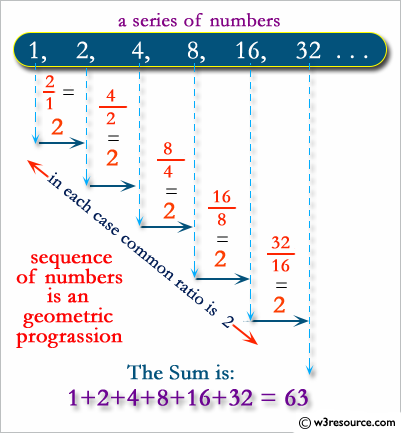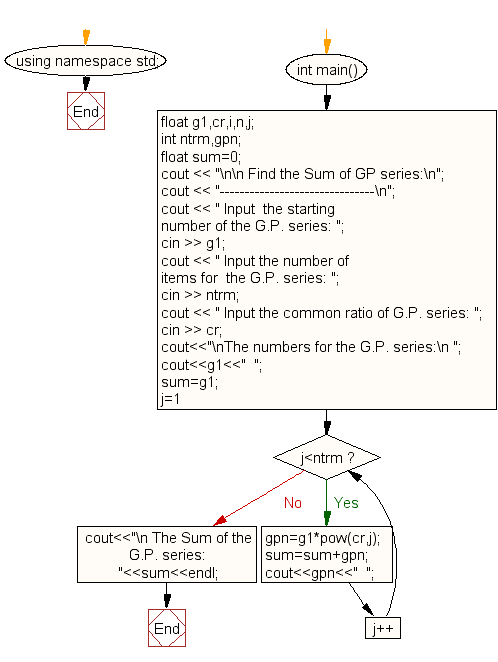﻿ C++ Exercises: Find the Sum of GP series - w3resource

# C++ Exercises: Find the Sum of GP series

## C++ For Loop: Exercise-32 with Solution

Write a C++ program to find the sum of the GP series.

Visual Presentation:Sample Solution:-

C++ Code :

``````#include <iostream> // Include the input/output stream library
#include <math.h>   // Include the math library for mathematical functions
using namespace std; // Using standard namespace

int main() // Main function where the execution of the program starts
{
float g1, cr, i, n, j; // Declare float variables g1, cr, i, n, j
int ntrm, gpn; // Declare integer variables ntrm and gpn
float sum = 0; // Declare and initialize a float variable sum to 0

// Display message asking for input
cout << "\n\n Find the Sum of GP series:\n";
cout << "-------------------------------\n";
cout << " Input  the starting number of the G.P. series: ";
cin >> g1; // Read input for the starting number of the G.P. series

cout << " Input the number of items for  the G.P. series: ";
cin >> ntrm; // Read input for the number of items for the G.P. series

cout << " Input the common ratio of G.P. series: ";
cin >> cr; // Read input for the common ratio of the G.P. series

/*-------- generate G.P. series ---------------*/
cout << "\nThe numbers for the G.P. series:\n ";
cout << g1 << "  "; // Display the starting number of the G.P. series
sum = g1; // Set sum to the starting number

// Loop to generate and display the G.P. series terms
for (j = 1; j < ntrm; j++) {
gpn = g1 * pow(cr, j); // Calculate each term of the G.P. series
sum = sum + gpn; // Add the term to the sum
cout << gpn << "  "; // Display the generated term
}
/*-------- End of G.P. series generate ---------------*/

cout << "\n The Sum of the G.P. series:  " << sum << endl; // Display the sum of the G.P. series
}

``````

Sample Output:

``` Find the Sum of GP series:
-------------------------------
Input  the starting number of the G.P. series: 3
Input the number of items for  the G.P. series: 5
Input the common ratio of G.P. series: 2

The numbers for the G.P. series:
3  6  12  24  48
The Sum of the G.P. series:  93
```

Flowchart:C++ Code Editor: